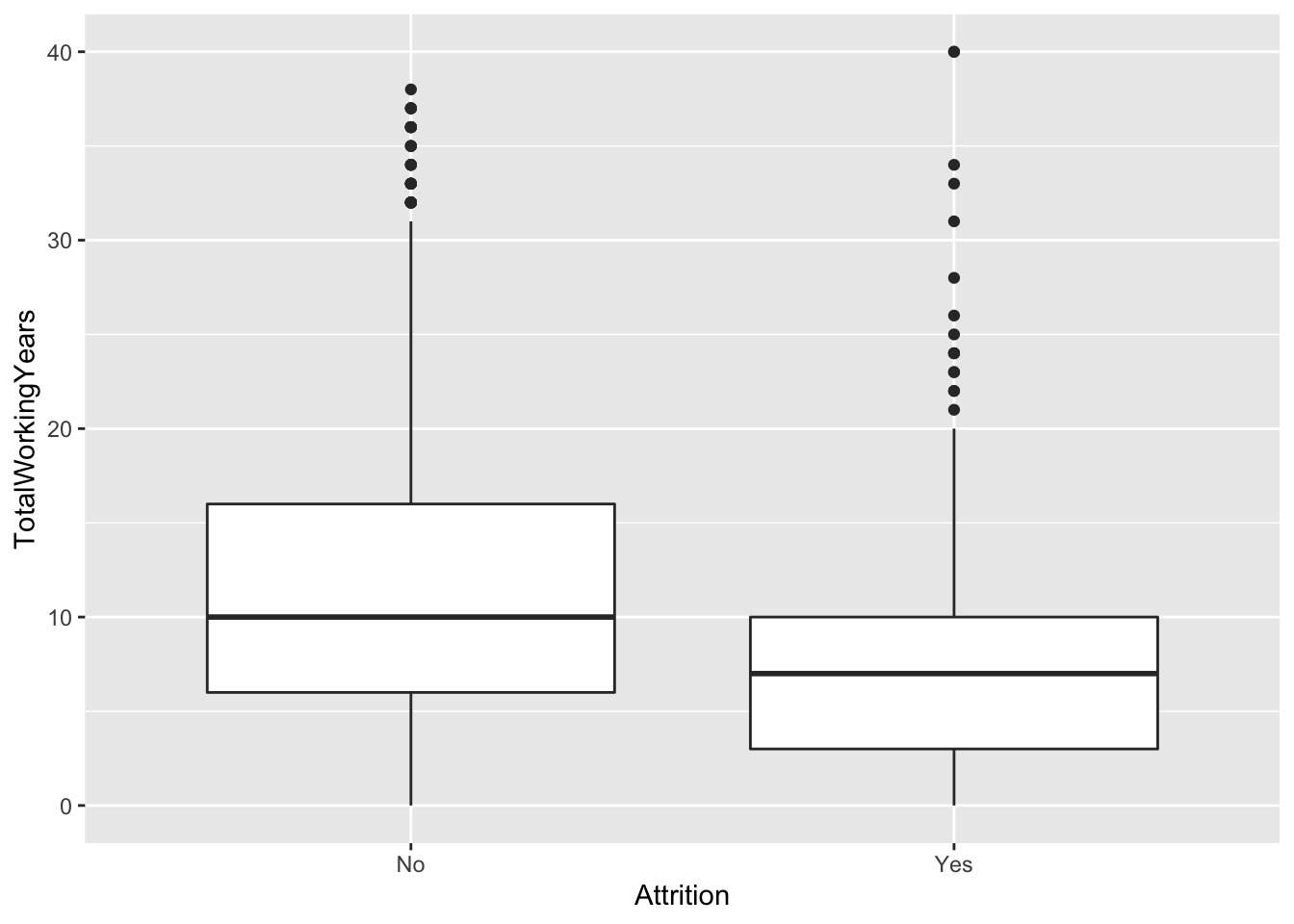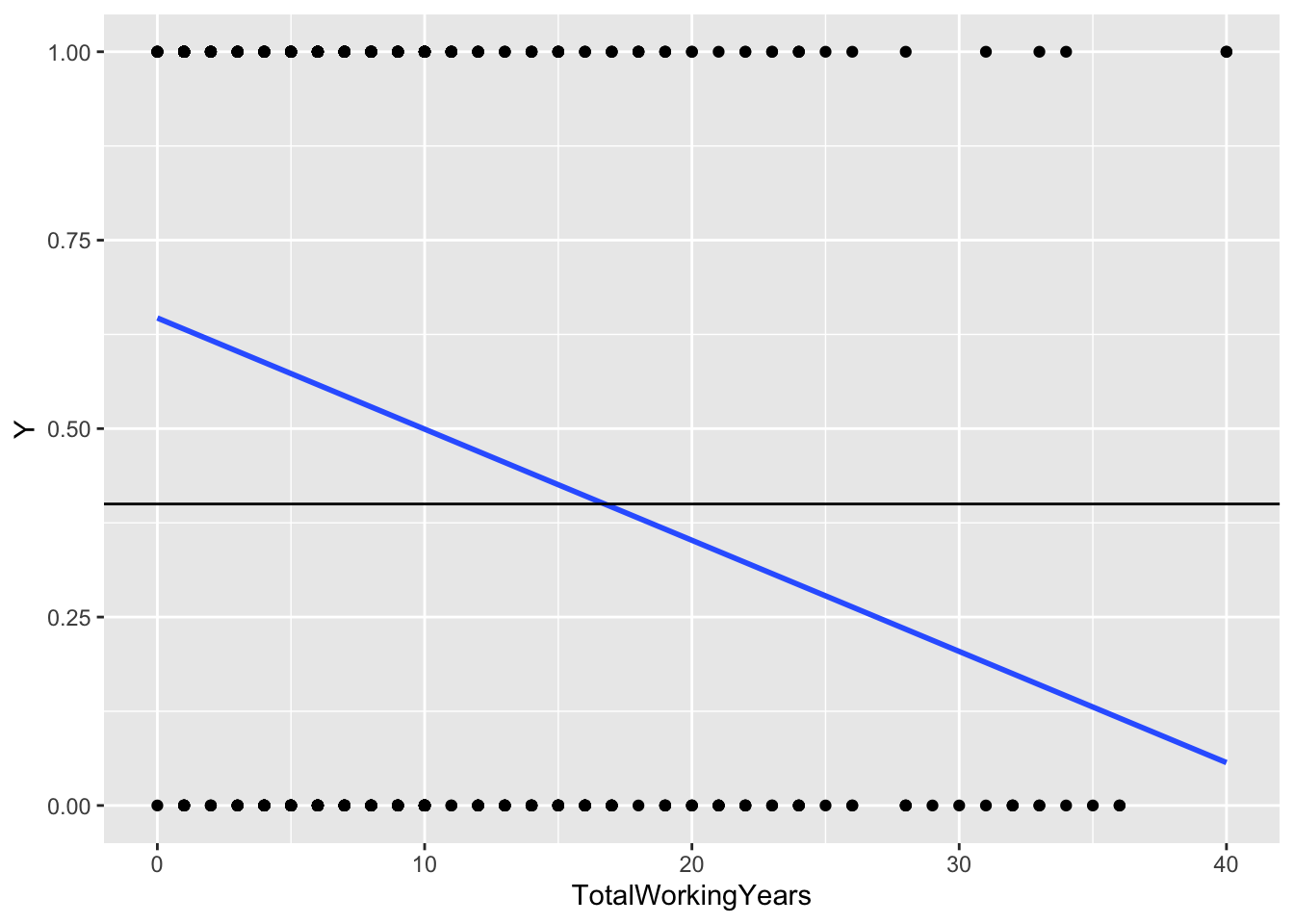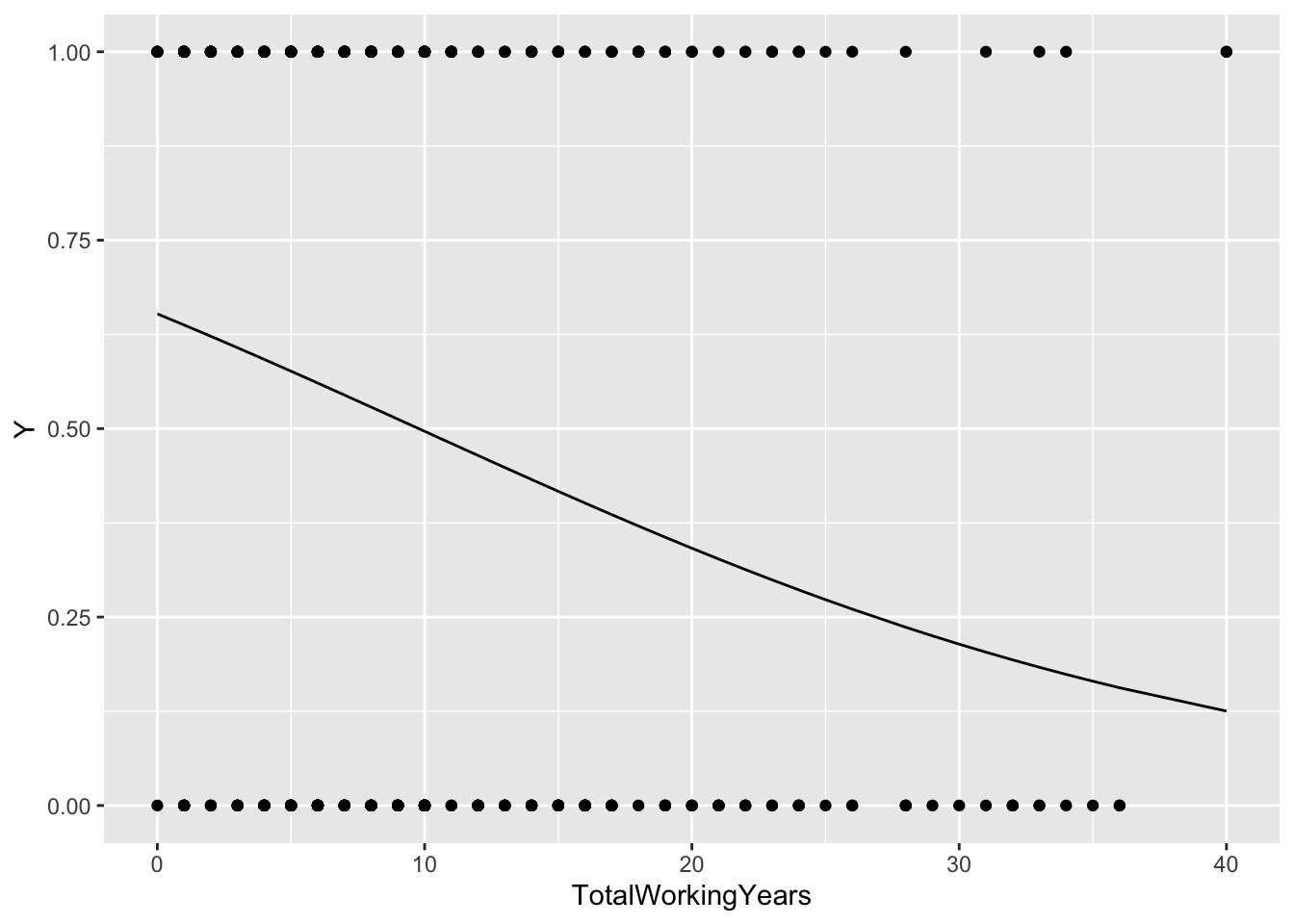## Categorical outcome data

Look at the data

head(attrition)
##   Age Attrition    BusinessTravel DailyRate           Department
## 1  41       Yes     Travel_Rarely      1102                Sales
## 2  49        No Travel_Frequently       279 Research_Development
## 4  37       Yes     Travel_Rarely      1373 Research_Development
## 5  33        No Travel_Frequently      1392 Research_Development
## 7  27        No     Travel_Rarely       591 Research_Development
## 8  32        No Travel_Frequently      1005 Research_Development
##   DistanceFromHome     Education EducationField EnvironmentSatisfaction Gender
## 1                1       College  Life_Sciences                  Medium Female
## 2                8 Below_College  Life_Sciences                    High   Male
## 4                2       College          Other               Very_High   Male
## 5                3        Master  Life_Sciences               Very_High Female
## 7                2 Below_College        Medical                     Low   Male
## 8                2       College  Life_Sciences               Very_High   Male
##   HourlyRate JobInvolvement JobLevel               JobRole JobSatisfaction
## 1         94           High        2       Sales_Executive       Very_High
## 2         61         Medium        2    Research_Scientist          Medium
## 4         92         Medium        1 Laboratory_Technician            High
## 5         56           High        1    Research_Scientist            High
## 7         40           High        1 Laboratory_Technician          Medium
## 8         79           High        1 Laboratory_Technician       Very_High
##   MaritalStatus MonthlyIncome MonthlyRate NumCompaniesWorked OverTime
## 1        Single          5993       19479                  8      Yes
## 2       Married          5130       24907                  1       No
## 4        Single          2090        2396                  6      Yes
## 5       Married          2909       23159                  1      Yes
## 7       Married          3468       16632                  9       No
## 8        Single          3068       11864                  0       No
##   PercentSalaryHike PerformanceRating RelationshipSatisfaction StockOptionLevel
## 1                11         Excellent                      Low                0
## 2                23       Outstanding                Very_High                1
## 4                15         Excellent                   Medium                0
## 5                11         Excellent                     High                0
## 7                12         Excellent                Very_High                1
## 8                13         Excellent                     High                0
##   TotalWorkingYears TrainingTimesLastYear WorkLifeBalance YearsAtCompany
## 1                 8                     0             Bad              6
## 2                10                     3          Better             10
## 4                 7                     3          Better              0
## 5                 8                     3          Better              8
## 7                 6                     3          Better              2
## 8                 8                     2            Good              7
## 1                  4                       0                    5
## 2                  7                       1                    7
## 4                  0                       0                    0
## 5                  7                       3                    0
## 7                  2                       2                    2
## 8                  7                       3                    6

Compare the distribution of a numeric predictor variable between the two outcome classes

ggplot(attrition, aes(Attrition, TotalWorkingYears)) +
geom_boxplot()Test for difference in means

t.test(TotalWorkingYears ~ Attrition, data = attrition)
##
##  Welch Two Sample t-test
##
## data:  TotalWorkingYears by Attrition
## t = 7.0192, df = 350.88, p-value = 1.16e-11
## alternative hypothesis: true difference in means is not equal to 0
## 95 percent confidence interval:
##  2.604401 4.632019
## sample estimates:
##  mean in group No mean in group Yes
##         11.862936          8.244726

Check class balance:

attrition %>% count(Attrition) 
##   Attrition    n
## 1        No 1233
## 2       Yes  237
table(attrition$Attrition) # base R ## ## No Yes ## 1233 237 Create a balanced dataset with the same number of observations in both classes attr_No <- attrition %>% filter(Attrition == "No") %>% sample_n(size = 237) attr_Yes <- attrition %>% filter(Attrition == "Yes") attr <- rbind(attr_No, attr_Yes) # or attr <- attrition %>% group_by(Attrition) %>% slice_sample(n = 237) # transform outcome to numeric 0-1 nattr <- attr %>% mutate(Y = as.numeric(Attrition) - 1) %>% select(Y, TotalWorkingYears) ## Adding missing grouping variables: Attrition ## Classification: linear regression? Plot linear regression line, change the threshold ggplot(nattr, aes(x = TotalWorkingYears, y = Y)) + geom_point() + geom_smooth(method = "lm", se = FALSE) + geom_hline(yintercept = .4) # or e.g. mean(nattr$Y)
## geom_smooth() using formula 'y ~ x'Problems:

• Can predict outside 0-1 range
• Not directly interpretable as probabilities

### Thresholding ideas

Choose a threshold/cutoff value for predictor $$X$$, say $$c$$, and then classify

• $$\hat Y = 1$$ if $$X \geq c$$
• $$\hat Y = 0$$ otherwise

Or if the association is negative, change the sign

As we vary $$c$$, we trade-off between kinds of errors: false positives and false negatives

In the simple case with thresholding one predictor, the classification/decision rules are all equivalent whether we use linear regression or logistic regression (as long as the fitted relationship is monotone)

For multiple regression–when we have more predictors–we can then transform a numeric prediction from the model $$\hat Y$$ to a classification by using a threshold rule on the scale of the predictions (instead of on the scale of one predictor as before)

• $$\hat Y = 1$$ if $$x^T \hat \beta \geq c$$
• $$\hat Y = 0$$ otherwise

## Logistic regression

model_glm <- glm(Y ~ TotalWorkingYears, data = nattr, family = "binomial")
summary(model_glm)
##
## Call:
## glm(formula = Y ~ TotalWorkingYears, family = "binomial", data = nattr)
##
## Deviance Residuals:
##     Min       1Q   Median       3Q      Max
## -1.4534  -1.1713   0.1708   1.1025   2.0385
##
## Coefficients:
##                   Estimate Std. Error z value Pr(>|z|)
## (Intercept)        0.62893    0.16041   3.921 8.83e-05 ***
## TotalWorkingYears -0.06432    0.01369  -4.697 2.64e-06 ***
## ---
## Signif. codes:  0 '***' 0.001 '**' 0.01 '*' 0.05 '.' 0.1 ' ' 1
##
## (Dispersion parameter for binomial family taken to be 1)
##
##     Null deviance: 657.10  on 473  degrees of freedom
## Residual deviance: 632.16  on 472  degrees of freedom
## AIC: 636.16
##
## Number of Fisher Scoring iterations: 4
augment(model_glm, type.predict = "response") %>%
ggplot(aes(TotalWorkingYears, Y)) +
geom_point() +
geom_line(aes(y = .fitted))### Modeling assumption

$\text{logit}[P(Y = 1|X)] = \beta_0 + \beta_1 X$

$P(Y = 1|X) = \frac{e^{\beta_0 + \beta_1 X}}{1 + e^{\beta_0 + \beta_1 X}}$

### Interpreting coefficients

exp(coef(model_glm))
##       (Intercept) TotalWorkingYears
##         1.8756090         0.9377032

### Inference

exp(confint(model_glm))
## Waiting for profiling to be done...
##                       2.5 %   97.5 %
## (Intercept)       1.3745869 2.580038
## TotalWorkingYears 0.9120732 0.962495

Model evaluation measures

glance(model_glm)
## # A tibble: 1 x 8
##   null.deviance df.null logLik   AIC   BIC deviance df.residual  nobs
##           <dbl>   <int>  <dbl> <dbl> <dbl>    <dbl>       <int> <int>
## 1          657.     473  -316.  636.  644.     632.         472   474

Diagnostic plots: can do this but less common, harder to interpret “deviance residuals”

(Warning: residual plots almost always show “patterns”, can’t be interpreted the same way as for linear regression)

## Balance and (re)calibration

What portion of data are classified using a given cutoff for the predicted probability?

mean(predict(model_glm, type = "response") > 0.5)
##  0.5611814

Try different cutoffs

c(.3, .4, .5, .6, 7) %>%
map_dbl(function(cutoff) mean(predict(model_glm, type = "response") > cutoff))
##  0.9219409 0.8417722 0.5611814 0.1687764 0.0000000

Tabulate a confusion matrix using dplyr functions

conf_mat <- function(fitted_glm, cutoff = .5) {
augment(fitted_glm, type.predict = "response") %>%
mutate(Yhat = as.numeric(.fitted > cutoff)) %>%
count(Y, Yhat)
}
conf_mat(model_glm)
## # A tibble: 4 x 3
##       Y  Yhat     n
##   <dbl> <dbl> <int>
## 1     0     0   128
## 2     0     1   109
## 3     1     0    80
## 4     1     1   157

Try another cutoff

conf_mat(model_glm, .6)
## # A tibble: 4 x 3
##       Y  Yhat     n
##   <dbl> <dbl> <int>
## 1     0     0   220
## 2     0     1    17
## 3     1     0   174
## 4     1     1    63

## Multiple regression model

(Complete outside of class time unless time permits)

Pick a few predictors and repeat the above

library(GGally)
## Registered S3 method overwritten by 'GGally':
##   method from
##   +.gg   ggplot2
options(warnings= -1)
attrition %>%
select(Attrition, Age, Gender, TotalWorkingYears,
DistanceFromHome, JobSatisfaction) %>%
ggpairs(progress = FALSE, lower = list(bins = 20))
## stat_bin() using bins = 30. Pick better value with binwidth.
## stat_bin() using bins = 30. Pick better value with binwidth.
## stat_bin() using bins = 30. Pick better value with binwidth.
## stat_bin() using bins = 30. Pick better value with binwidth.
## stat_bin() using bins = 30. Pick better value with binwidth.
## stat_bin() using bins = 30. Pick better value with binwidth.
## stat_bin() using bins = 30. Pick better value with binwidth.
## stat_bin() using bins = 30. Pick better value with binwidth.
## stat_bin() using bins = 30. Pick better value with binwidth.